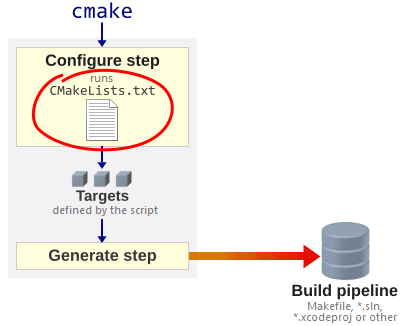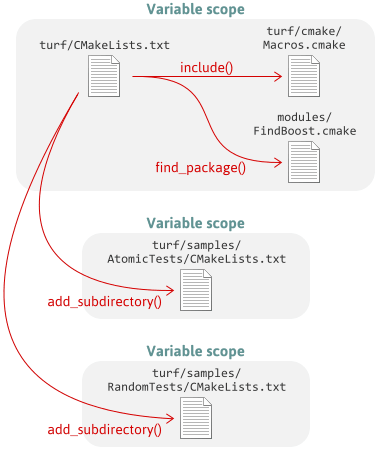# Learn CMake's Scripting Language in 15 Minutes

As explained in my previous post, every CMake-based project must contain a script named CMakeLists.txt. This script defines targets, but it can also do a lot of other things, such as finding third-party libraries or generating C++ header files. CMake scripts have a lot of flexibility.Every time you integrate an external library, and often when adding support for another platform, you’ll need to edit the script. I spent a long time editing CMake scripts without really understanding the language, as the documentation is quite scattered, but eventually, things clicked. The goal of this post is to get you to the same point as quickly as possible.

This post won’t cover all of CMake’s built-in commands, as there are hundreds, but it is a fairly complete guide to the syntax and programming model of the language.

## Hello World

If you create a file named hello.txt with the following contents:

message("Hello world!")         # A message to print


…you can run it from the command line using cmake -P hello.txt. (The -P option runs the given script, but doesn’t generate a build pipeline.) As expected, it prints “Hello world!”.

$cmake -P hello.txt Hello world!  ## All Variables Are Strings In CMake, every variable is a string. You can substitute a variable inside a string literal by surrounding it with ${}. This is called a variable reference. Modify hello.txt as follows:

message("Hello ${NAME}!") # Substitute a variable into the message  Now, if we define NAME on the cmake command line using the -D option, the script will use it: $ cmake -DNAME=Newman -P hello.txt
Hello Newman!


When a variable is undefined, it defaults to an empty string:

$cmake -P hello.txt Hello !  To define a variable inside a script, use the set command. The first argument is the name of the variable to assign, and the second argument is its value: set(THING "funk") message("We want the${THING}!")


Quotes around arguments are optional, as long as there are no spaces or variable references in the argument. For example, I could have written set("THING" funk) in the first line above – it would have been equivalent. For most CMake commands (except if and while, described below), the choice of whether to quote such arguments is simply a matter of style. When the argument is the name of a variable, I tend not to use quotes.

## You Can Simulate a Data Structure using Prefixes

CMake does not have classes, but you can simulate a data structure by defining a group of variables with names that begin with the same prefix. You can then look up variables in that group using nested ${} variable references. For example, the following script will print “John Smith lives at 123 Fake St.”: set(JOHN_NAME "John Smith") set(JOHN_ADDRESS "123 Fake St") set(PERSON "JOHN") message("${${PERSON}_NAME} lives at${${PERSON}_ADDRESS}.")  You can even use variable references in the name of the variable to set. For example, if the value of PERSON is still “JOHN”, the following will set the variable JOHN_NAME to “John Goodman”: set(${PERSON}_NAME "John Goodman")


## Every Statement is a Command

In CMake, every statement is a command that takes a list of string arguments and has no return value. Arguments are separated by (unquoted) spaces. As we’ve already seen, the set command defines a variable at file scope.

As another example, CMake has a math command that performs arithmetic. The first argument must be EXPR, the second argument is the name of the variable to assign, and the third argument is the expression to evaluate – all strings. Note that on the third line below, CMake substitutes the string value of MY_SUM into the enclosing argument before passing the argument to math.

math(EXPR MY_SUM "1 + 1")                   # Evaluate 1 + 1; store result in MY_SUM
message("The sum is ${MY_SUM}.") math(EXPR DOUBLE_SUM "${MY_SUM} * 2")       # Multiply by 2; store result in DOUBLE_SUM
message("Double that is ${DOUBLE_SUM}.")  There’s a CMake command for just about anything you’ll need to do. The string command lets you perform advanced string manipulation, including regular expression replacement. The file command can read or write files, or manipulate filesystem paths. ## Flow Control Commands Even flow control statements are commands. The if/endif commands execute the enclosed commands conditionally. Whitespace doesn’t matter, but it’s common to indent the enclosed commands for readablity. The following checks whether CMake’s built-in variable WIN32 is set: if(WIN32) message("You're running CMake on Windows.") endif()  CMake also has while/endwhile commands which, as you might expect, repeat the enclosed commands as long as the condition is true. Here’s a loop that prints all the Fibonacci numbers up to one million: set(A "1") set(B "1") while(A LESS "1000000") message("${A}")                 # Print A
math(EXPR T "${A} +${B}")      # Add the numeric values of A and B; store result in T
set(A "${B}") # Assign the value of B to A set(B "${T}")                   # Assign the value of T to B
endwhile()


CMake’s if and while conditions aren’t written the same way as in other languages. For example, to perform a numeric comparison, you must specify LESS as a string argument, as shown above. The documentation explains how to write a valid condition.

if and while are different from other CMake commands in that if the name of a variable is specified without quotes, the command will use the variable’s value. In the above code, I took advantage of that behavior by writing while(A LESS "1000000") instead of while("${A}" LESS "1000000") – both forms are equivalent. Other CMake commands don’t do that. ## Lists are Just Semicolon-Delimited Strings CMake has a special substitution rule for unquoted arguments. If the entire argument is a variable reference without quotes, and the variable’s value contains semicolons, CMake will split the value at the semicolons and pass multiple arguments to the enclosing command. For example, the following passes three arguments to math: set(ARGS "EXPR;T;1 + 1") math(${ARGS})                                   # Equivalent to calling math(EXPR T "1 + 1")


On the other hand, quoted arguments are never split into multiple arguments, even after substitution. CMake always passes a quoted string as a single argument, leaving semicolons intact:

set(ARGS "EXPR;T;1 + 1")
message("${ARGS}") # Prints: EXPR;T;1 + 1  If more than two arguments are passed to the set command, they are joined by semicolons, then assigned to the specified variable. This effectively creates a list from the arguments: set(MY_LIST These are separate arguments) message("${MY_LIST}")                           # Prints: These;are;separate;arguments


You can manipulate such lists using the list command:

set(MY_LIST These are separate arguments)
list(REMOVE_ITEM MY_LIST "separate")            # Removes "separate" from the list
message("${MY_LIST}") # Prints: These;are;arguments  The foreach/endforeach command accepts multiple arguments. It iterates over all arguments except the first, assigning each one to the named variable: foreach(ARG These are separate arguments) message("${ARG}")                           # Prints each word on a separate line
endforeach()


You can iterate over a list by passing an unquoted variable reference to foreach. As with any other command, CMake will split the variable’s value and pass multiple arguments to the command:

foreach(ARG ${MY_LIST}) # Splits the list; passes items as arguments message("${ARG}")                           # Prints each item on a separate line
endforeach()


## Functions Run In Their Own Scope; Macros Don’t

In CMake, you can use a pair of function/endfunction commands to define a function. Here’s one that doubles the numeric value of its argument, then prints the result:

function(doubleIt VALUE)
math(EXPR RESULT "${VALUE} * 2") message("${RESULT}")
endfunction()

doubleIt("4")                           # Prints: 8


Functions run in their own scope. None of the variables defined in a function pollute the caller’s scope. If you want to return a value, you can pass the name of a variable to your function, then call the set command with the special argument PARENT_SCOPE:

function(doubleIt VARNAME VALUE)
math(EXPR RESULT "${VALUE} * 2") set(${VARNAME} "${RESULT}" PARENT_SCOPE) # Set the named variable in caller's scope endfunction() doubleIt(RESULT "4") # Tell the function to set the variable named RESULT message("${RESULT}")                    # Prints: 8


Similarly, a pair of macro/endmacro commands defines a macro. Unlike functions, macros run in the same scope as their caller. Therefore, all variables defined inside a macro are set in the caller’s scope. We can replace the previous function with the following:

macro(doubleIt VARNAME VALUE)
math(EXPR ${VARNAME} "${VALUE} * 2")        # Set the named variable in caller's scope
endmacro()

doubleIt(RESULT "4")                    # Tell the macro to set the variable named RESULT
message("${RESULT}") # Prints: 8  Both functions and macros accept an arbitrary number of arguments. Unnamed arguments are exposed to the function as a list, through a special variable named ARGN. Here’s a function that doubles every argument it receives, printing each one on a separate line: function(doubleEach) foreach(ARG${ARGN})                # Iterate over each argument
math(EXPR N "${ARG} * 2") # Double ARG's numeric value; store result in N message("${N}")                 # Print N
endforeach()
endfunction()

doubleEach(5 6 7 8)                     # Prints 10, 12, 14, 16 on separate lines


## Including Other Scripts

CMake variables are defined at file scope. The include command executes another CMake script in the same scope as the calling script. It’s a lot like the #include directive in C/C++. It’s typically used to define a common set of functions or macros in the calling script. It uses the variable CMAKE_MODULE_PATH as a search path.

The find_package command looks for scripts of the form Find*.cmake and also runs them in the same scope. Such scripts are often used to help find external libraries. For example, if there is a file named FindSDL2.cmake in the search path, find_package(SDL2) is equivalent to include(FindSDL2.cmake). (Note that there are several ways to use the find_package command – this is just one of them.)

CMake’s add_subdirectory command, on the other hand, creates a new scope, then executes the script named CMakeLists.txt from the specified directory in that new scope. You typically use it to add another CMake-based subproject, such as a library or executable, to the calling project. The targets defined by the subproject are added to the build pipeline unless otherwise specified. None of the variables defined in the subproject’s script will pollute the parent’s scope unless the set command’s PARENT_SCOPE option is used.

As an example, here are some of the scripts involved when you run CMake on the Turf project:## Getting and Setting Properties

A CMake script defines targets using the add_executable, add_library or add_custom_target commands. Once a target is created, it has properties that you can manipulate using the get_property and set_property commands. Unlike variables, targets are visible in every scope, even if they were defined in a subdirectory. All target properties are strings.

add_executable(MyApp "main.cpp")        # Create a target named MyApp

# Get the target's SOURCES property and assign it to MYAPP_SOURCES
get_property(MYAPP_SOURCES TARGET MyApp PROPERTY SOURCES)

message("\${MYAPP_SOURCES}")             # Prints: main.cpp


Other target properties include LINK_LIBRARIES, INCLUDE_DIRECTORIES and COMPILE_DEFINITIONS. Those properties are modified, indirectly, by the target_link_libraries, target_include_directories and target_compile_definitions commands. At the end of the script, CMake uses those target properties to generate the build pipeline.

There are properties for other CMake entities, too. There is a set of directory properties at every file scope. There is a set of global properties that is accessible from all scripts. And there is a set of source file properties for every C/C++ source file.

Congratulations! You now know the CMake scripting language – or at least, it should be easier to understand large scripts using CMake’s command reference. Otherwise, the only thing missing from this guide, that I can think of, is generator expressions. Let me know if I forgot anything else!